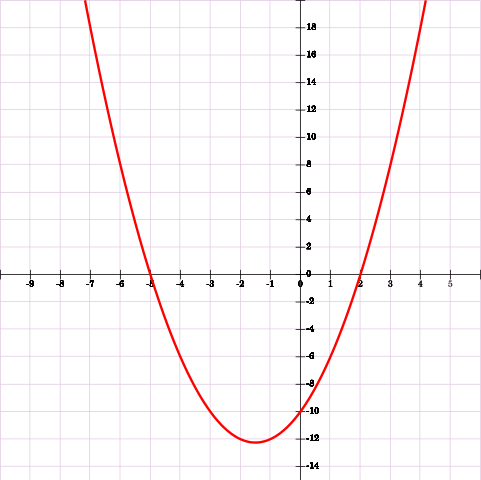I have a horrible memory. I don’t mean just that I misplace things or forget names; it takes a lot of effort to commit arbitrary facts, figures, dates, etc., to my long-term memory. So throughout my school years, most of my studying was for things like History, trying hard to remember dates and statistics that I would quickly eject from my mind after my next exam. I seldom had to study for Math or Science though, because I figured out something that worked for me there: learning how and why things work rather than just memorizing formulas. This worked well for those subjects, but I do remember stumbling in algebra when I was not able to factor quadratic functions. There was a handy Swiss army knife of sorts for this, of course, in the Quadratic Formula.

I avoided this formula as much as possible, usually by spending way too much time trying to guess the factors myself, or by converting from “standard form” to “vertex form”, or guessing, or skipping that question. This was almost entirely because I could not bring myself to memorize the formula. Call it laziness, or foolishness, or whatever you’d like.

Well, recently I decided to brush up on my math skills. After yet again encountering the need for this equation, I decided enough is enough. Since I can’t memorize the equation, I will instead learn where it comes from by deriving it from the standard form of a quadratic function. This is my attempt to do so.

To start, I’ll actually show both the beginning (the standard form):$ax^2 + bx + c = 0$

and the end (the Quadratic Equation):$x = \frac{-b \pm \sqrt{b^2 - 4ac}}{2a}$

The goal is to isolate$x$ from everything else, though doing so will get a bit tricky. First, I can subtract$c$ from both sides:$ax^2 + bx + c - c = 0 - c$$ax^2 + bx = -c$

Now I’ll divide both sides by$a$:$\frac{ax^2 + bx}{a} = -\frac{c}{a}$

Cleaning this up a bit, I end up with:$x^2 + \frac{bx}{a} = -\frac{c}{a}$

Now it looks like I’m getting somewhere, but where do I go from here? It sure would be nice if I could just convert the whole left side of the equation to a squared form… which I can with some simple (though not intuitive) addition on both sides of the equation. The key lies in understanding what to add to the left side of the equation to reach something in the form of$(x + n)^2$. Here is a generic way of describing how this works:

To go from$x^2 + nx$ to a squared quantity, I can take the linear coefficient (the$n$ in this example), divide it by$2$, then square the result:$x^2 + nx = 0$$x^2 + nx + (\frac{n}{2})^2 = 0 + (\frac{n}{2})^2$$x^2 + nx + \frac{n^2}{4} = \frac{n^2}{4}$$(x + \frac{n}{2})^2 = \frac{n^2}{4}$

As a better example, I’ll use an equation with real numbers:$x^2 + 8x = -16$$x^2 + 8x + (\frac{8}{2})^2 = -16 + (\frac{8}{2})^2$$x^2 + 8x + (4)^2 = -16 +(4)^2$$x^2 + 8x + 16 = 0$$(x + \frac{8}{2})^2 = 0$$(x + 4)^2 = 0$

So… bringing it back to the original goal, I can apply this same trick to my original equation to help make my square quantity:$x^2 + \frac{bx}{a} = -\frac{c}{a}$

My$n$ here is$\frac{b}{a}$, so, I can first divide it by$2$:$\frac{b}{a} * \frac{1}{2} = \frac{b}{2a}$

Now I can square that:$(\frac{b}{2a})^2 = \frac{b^2}{4a^2}$

and I have my value to add to both sides of my equation:$x^2 + \frac{bx}{a} + \frac{b^2}{4a^2} = -\frac{c}{a} + \frac{b^2}{4a^2}$

Now I can build a square quantity on the left:$(x + \frac{b}{2a})^2 = -\frac{c}{a} + \frac{b^2}{4a^2}$

Okay, I’m getting closer, but I don’t really like the right side. Let me combine the right side by reaching a common denominator. To do that, I’ll multiply by$\frac{4a}{4a}$, which is really just$1$:$(x + \frac{b}{2a})^2 = (-\frac{c}{a} * \frac{4a}{4a}) + \frac{b^2}{4a^2}$$(x + \frac{b}{2a})^2 = -\frac{4ac}{4a^2} + \frac{b^2}{4a^2}$$(x + \frac{b}{2a})^2 = \frac{b^2 - 4ac}{4a^2}$

Now, I can take the square root of both sides:$\sqrt{(x + \frac{b}{2a})^2} = \pm \sqrt{\frac{b^2 - 4ac}{4a^2}}$$x + \frac{b}{2a} = \pm \frac{\sqrt{b^2 - 4ac}}{\sqrt{4a^2}}$$x + \frac{b}{2a} = \pm \frac{\sqrt{b^2 - 4ac}}{2a}$

And the final step of isolating$x$:$x + \frac{b}{2a} - \frac{b}{2a} = \pm \frac{\sqrt{b^2 - 4ac}}{2a} - \frac{b}{2a}$$x = \frac{-b \pm \sqrt{b^2 - 4ac}}{2a}$

And there you have it.

The real question is, why is this useful? Well, for one, it is a good exercise to revive some old and atrophying math skills. It also helps me understand where the Quadratic Formula came from, and therefore helps me remember it. I hope this walk-through stimulates others out there as well.